# RC Car with laser turret!

Arduino RC car, using a joystick shield and NRF24l01 modules.

IntermediateFull instructions provided499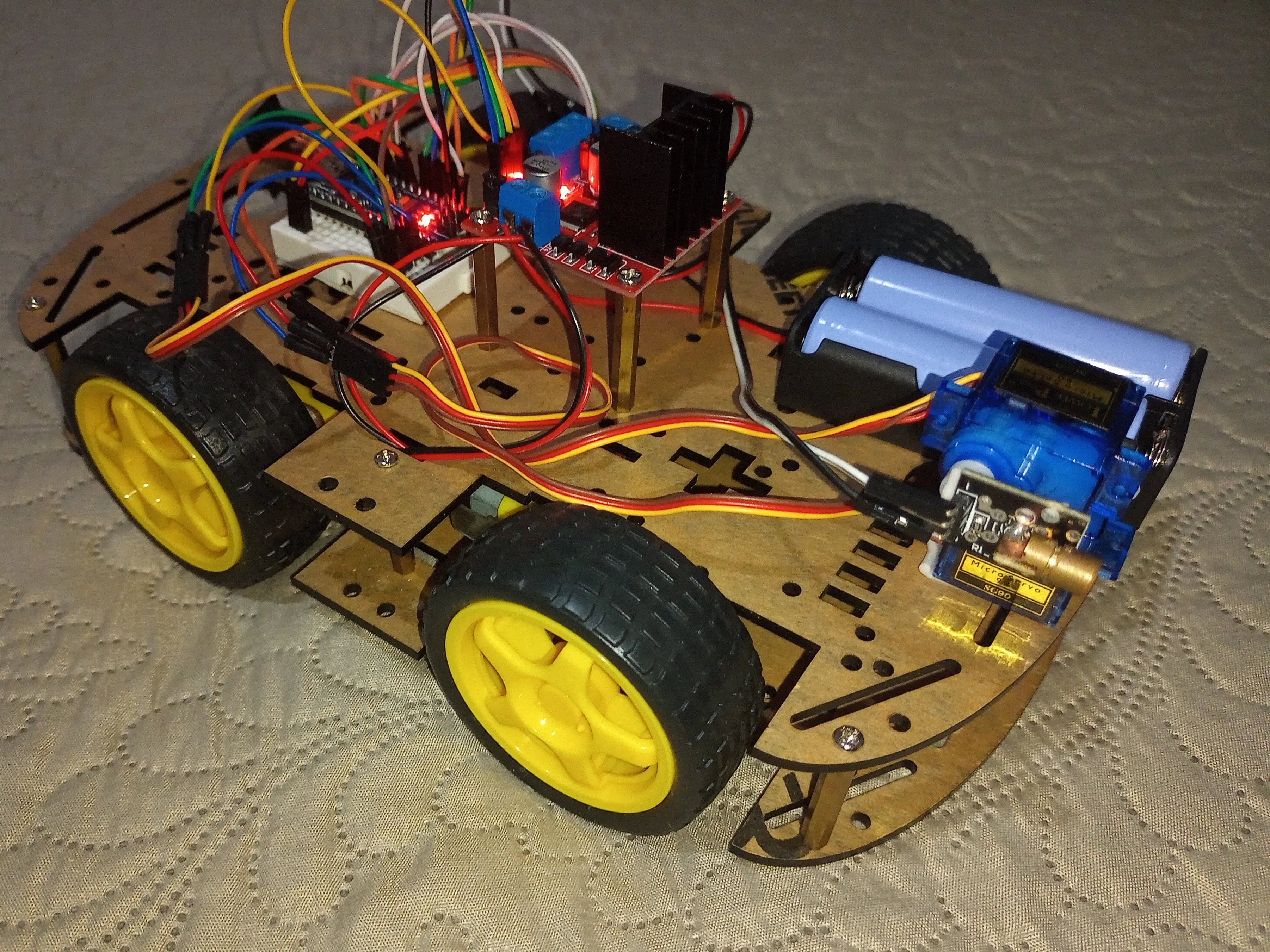## Things used in this project

### Hardware componentsArduino Nano R3 Car microcontroller
×1Arduino UNO Remote control controler
×1
 Funduino Joystick Shield V1.A Shield to Arduino Uno (RC)
×1
 nRF24 Module (Generic) NRF24L01+ module
×2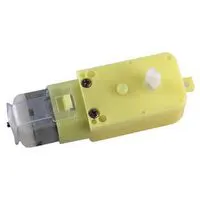DC Motor, 12 V Move the car
×4
 Plastic wheel for motor Wheels for motors
×1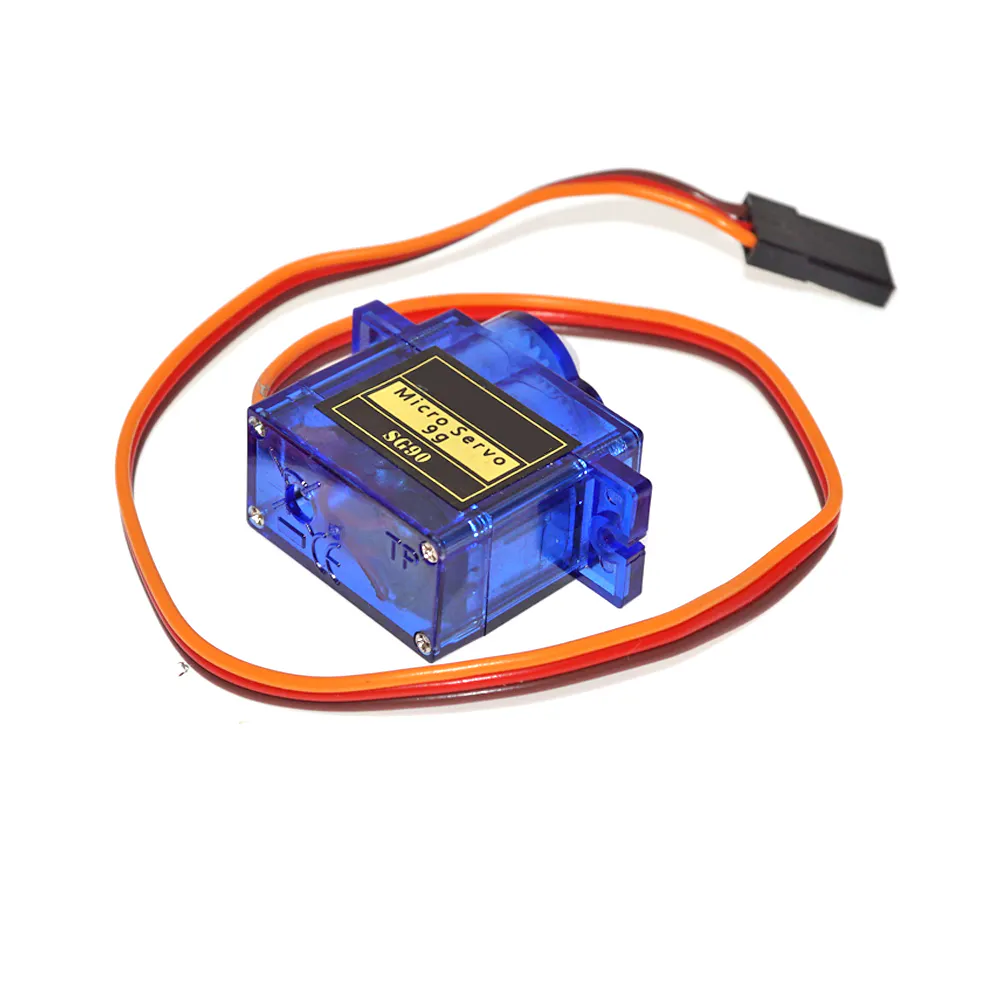SG90 Micro-servo motor Turret
×2
 Laser diode module Laser
×1
 4.2v 18650 Batteries Power source
×4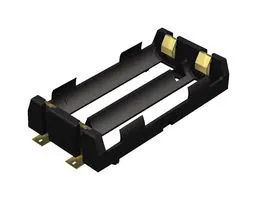Battery Holder, 18650 x 2 Hold 18650 batteries
×2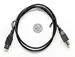USB-A to B Cable To upload the program to Arduino Uno
×1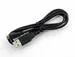USB-A to Micro-USB Cable To upload the program to Arduino Nano
×1Jumper wires (generic)
×6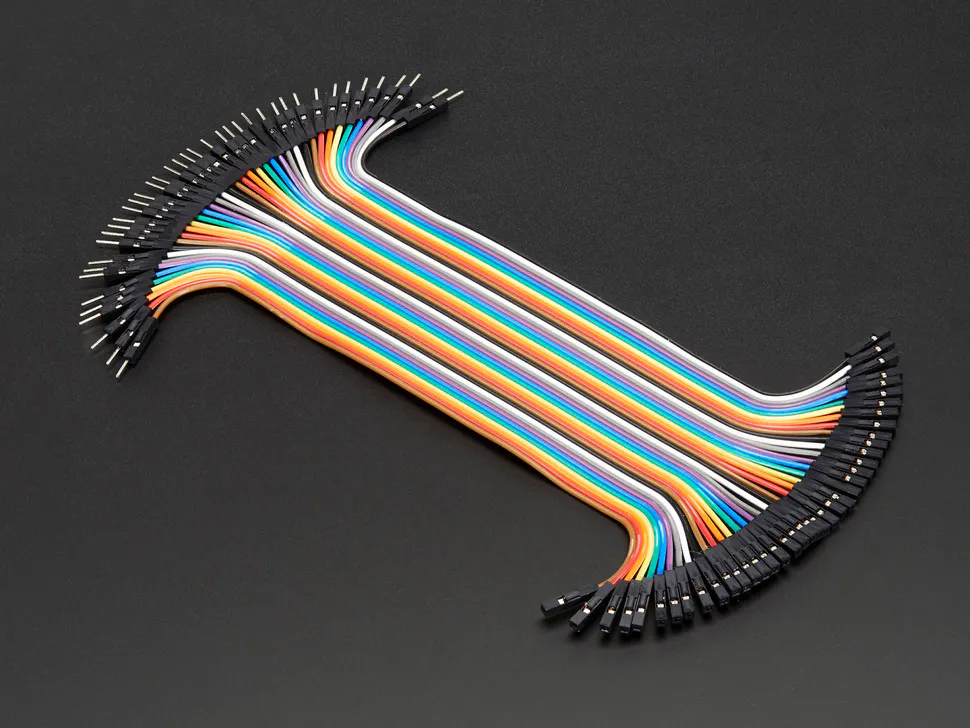Male/Female Jumper Wires
×15

### Software apps and online servicesArduino IDE

### Hand tools and fabrication machines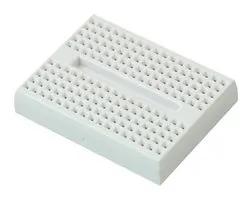## Schematics

### Transmitter circuit

Connections of joystick shield on Arduino. Battery connection.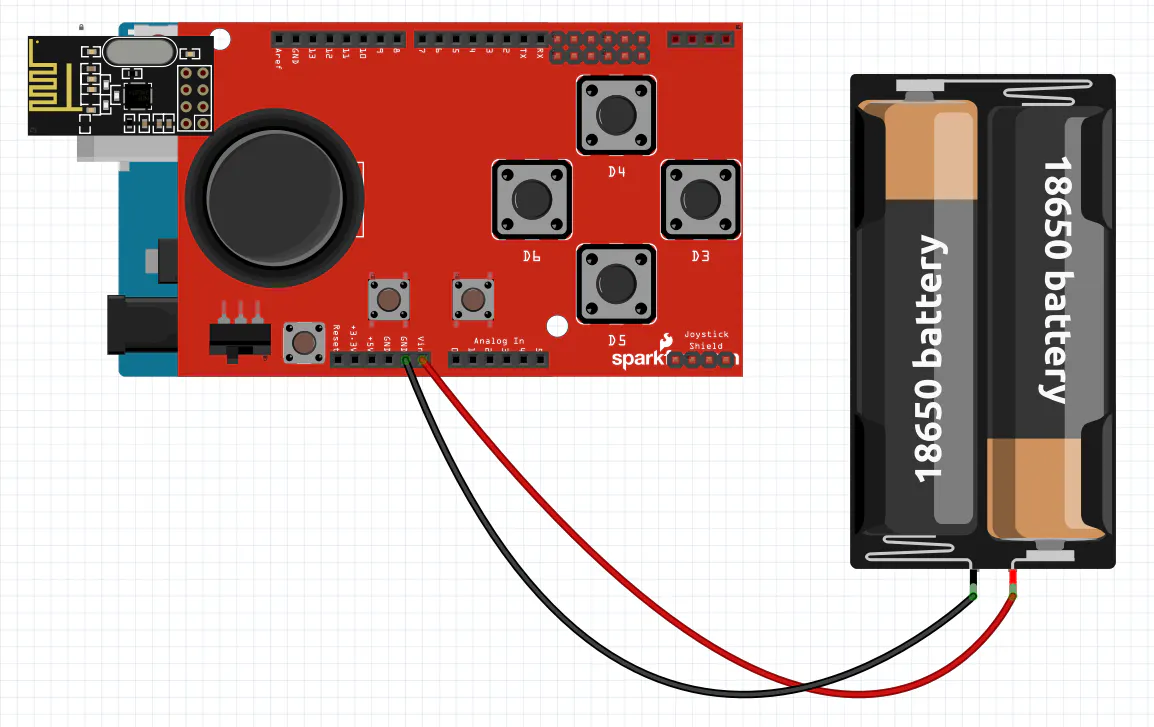Circuit of the RC car.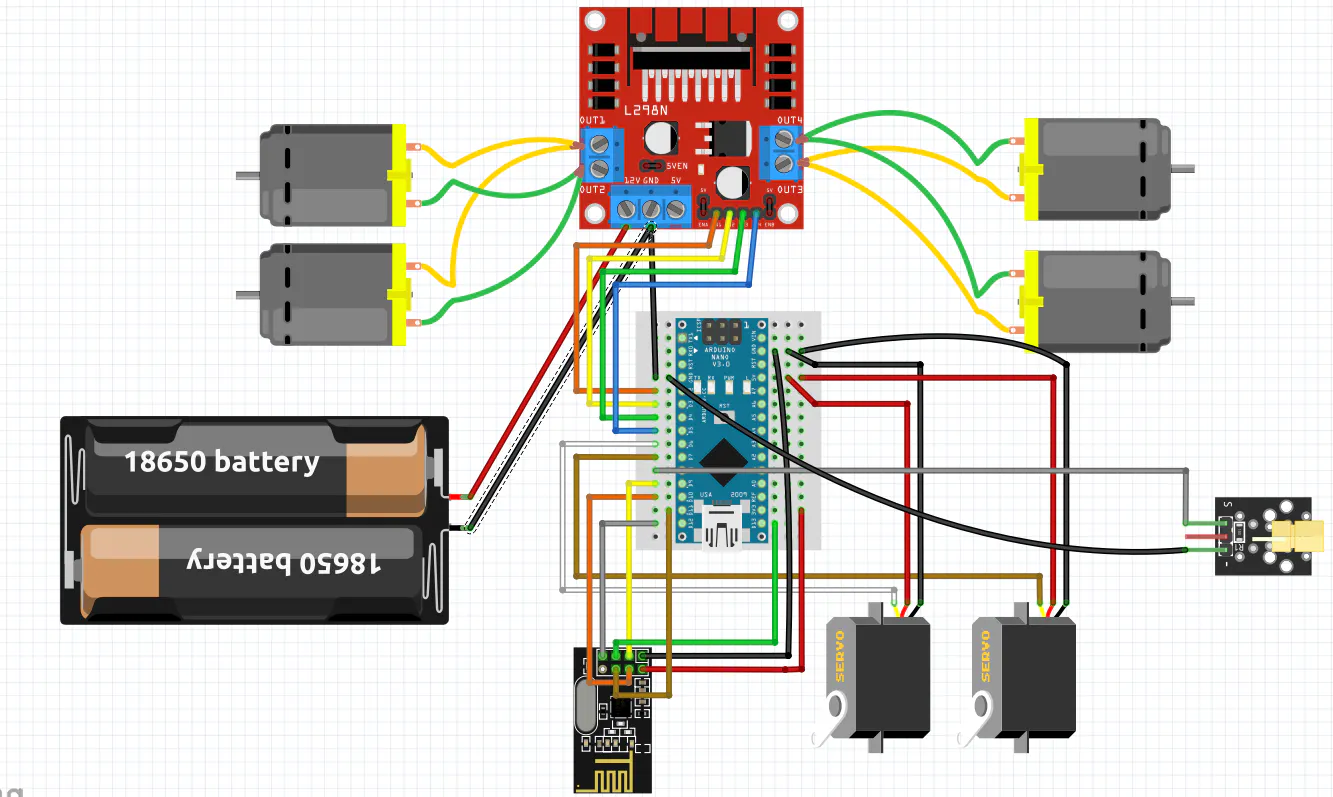## Code

### Transmitter code

C/C++
Transmitter code for the project
```//Include Libraries
#include <nRF24L01.h>
#include <RF24.h>
#include <SPI.h>
//Creates objects
#define A 2
#define B 3
#define C 4
#define D 5
#define E 6
int X = A0;
int Y = A1;

const uint64_t pipe = 0xE6E6E6E6E6E6;
char msg = "";

void setup() {
//Define buttons of the shield as Input
pinMode(A, INPUT);
pinMode(B, INPUT);
pinMode(C, INPUT);
pinMode(D, INPUT);
pinMode(E, INPUT);
digitalWrite(A, HIGH);
digitalWrite(B, HIGH);
digitalWrite(C, HIGH);
digitalWrite(D, HIGH);
digitalWrite(E, HIGH);
//Starts the RF module
}

void loop() {

if (digitalRead(A) == LOW) { //Moves the turret pointer up
char msg[] = "up";
}
if (digitalRead(C) == LOW) { //Moves the turret pointer down
char msg[] = "down";
}
if (digitalRead(B) == LOW) { //Moves the turret to left
char msg[] = "leftturret";
}
if (digitalRead(D) == LOW) { //Moves the turret to right
char msg[] = "rightturret";
}
if (digitalRead(E) == LOW) { //Shots
char msg[] = "shot";
}
if (analogRead(X) < 450) { //Turn left
char msg[] = "left";
}
if (analogRead(X) > 550) { //Turn right
char msg[] = "right";
}
if (analogRead(Y) > 550) { //Go forward
char msg[] = "forward";
}
if (analogRead(Y) < 450) { //Go backward
char msg[] = "backward";
}
else { //Nothing happens
char msg[] = "analgobut";
}
}
```

C/C++
```//include libraries
#include <nRF24L01.h>
#include <RF24.h>
#include <SPI.h>
#include <Servo.h>
//Create objects
int RightMotorF = 2;
int RightMotorB = 3;
int LeftMotorF = 4;
int LeftMotorB = 5;
int laser = 8;
Servo turret1;
Servo turret2;
int pos1 = 90;
int pos2 = 90;
const uint64_t pipe = 0xE6E6E6E6E6E6;
char data = "";

void setup() {
//Starts thee RF module
//Servo motor pins
turret1.attach(6);
turret2.attach(7);
//Define motor pins as output
pinMode(RightMotorB, OUTPUT);
pinMode(LeftMotorB, OUTPUT);
pinMode(RightMotorF, OUTPUT);
pinMode(LeftMotorF, OUTPUT);
//Define laser as output
pinMode(laser, OUTPUT);
}
void loop() {
String msg = "";
{
Serial.println(data);
msg = data;

if (msg == "forward") //Go forward
{
digitalWrite(RightMotorB, HIGH);
digitalWrite(LeftMotorB, HIGH);
digitalWrite(RightMotorF, LOW);
digitalWrite(LeftMotorF, LOW);

}
else if (msg == "backward") //Go backward
{
digitalWrite(RightMotorB, LOW);
digitalWrite(LeftMotorB, LOW);
digitalWrite(RightMotorF, HIGH);
digitalWrite(LeftMotorF, HIGH);
}
else if (msg == "left") //Turn left
{
digitalWrite(RightMotorB, LOW);
digitalWrite(LeftMotorB, HIGH);
digitalWrite(RightMotorF, LOW);
digitalWrite(LeftMotorF, LOW);
}
else if (msg == "right") //Turn right
{
digitalWrite(RightMotorB, HIGH);
digitalWrite(LeftMotorB, LOW);
digitalWrite(RightMotorF, LOW);
digitalWrite(LeftMotorF, LOW);
}
else if (msg == "rightturret") //Turret base turn right
{
pos1++;
}
else if (msg == "leftturret") { //Turret base turn left
pos1--;
}
else if (msg == "up") //Turret pointer go up
{
pos2++;
}
else if (msg == "down") {//Turret pointer go down
pos2--;
}
else if (msg == "shot") { //Shots laser
digitalWrite(laser, HIGH);
delay(50);
digitalWrite(laser, LOW);
delay(50);
}
else if (msg == "analgobut") //If nothing happens
{
digitalWrite(RightMotorB, LOW);
digitalWrite(LeftMotorB, LOW);
digitalWrite(RightMotorF, LOW);
digitalWrite(LeftMotorF, LOW);
}